# Probability solutions inter maths 2a

Intermediate mathematics IIA Probability solutions for text book exercises 9(a), 9(b) and 9(c).

These are very easy to understand. First you study the text book well.

Practice the example problems and solutions.

Observe the solutions and try them in your own method

You can see the solutions for maths IIA

Complex numbers textbook exercises solutions

De Moivre’s Theorem textbook solutions

Exercise 3(b)

Exercise 3(c)

You can see solutions for II B

1. Circle

3. Parabola

4. Ellipse

You can see the solutions for first year maths 1A and 1B for examination purpose

Inter maths 1A solutions

3. Matrices

You can also see the solutions for 1b

1. Locus

The straight line sa

Straight lines la

# Exercise 9(a), 9(b) and 9(c) Intermediate Mathematics 2nd year

Probability – Exercise 9(a)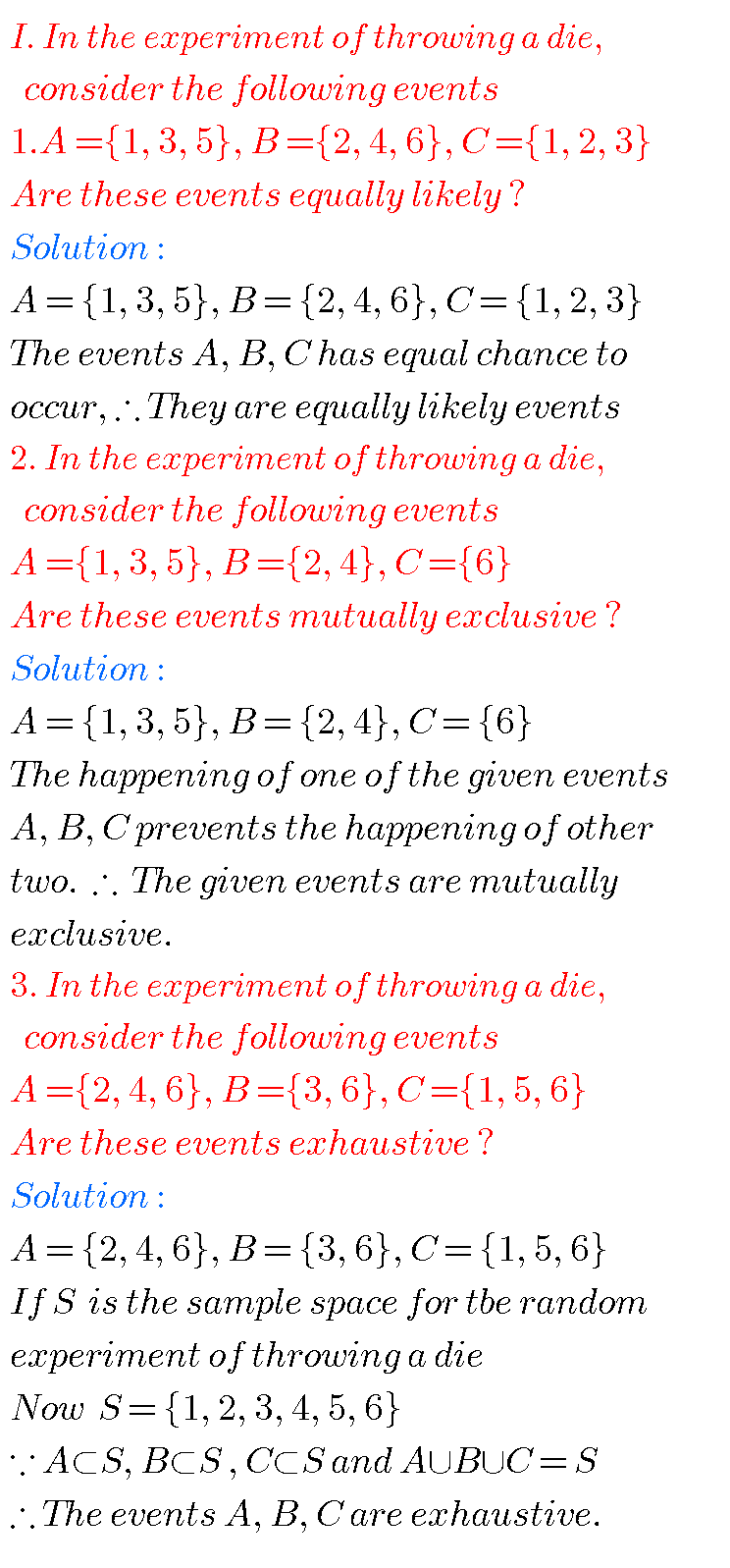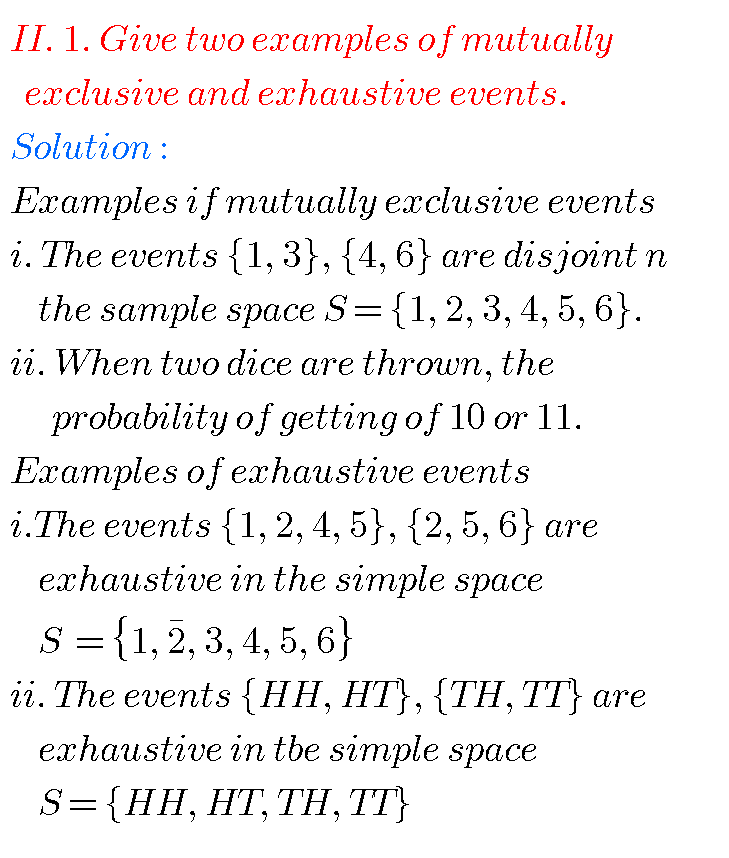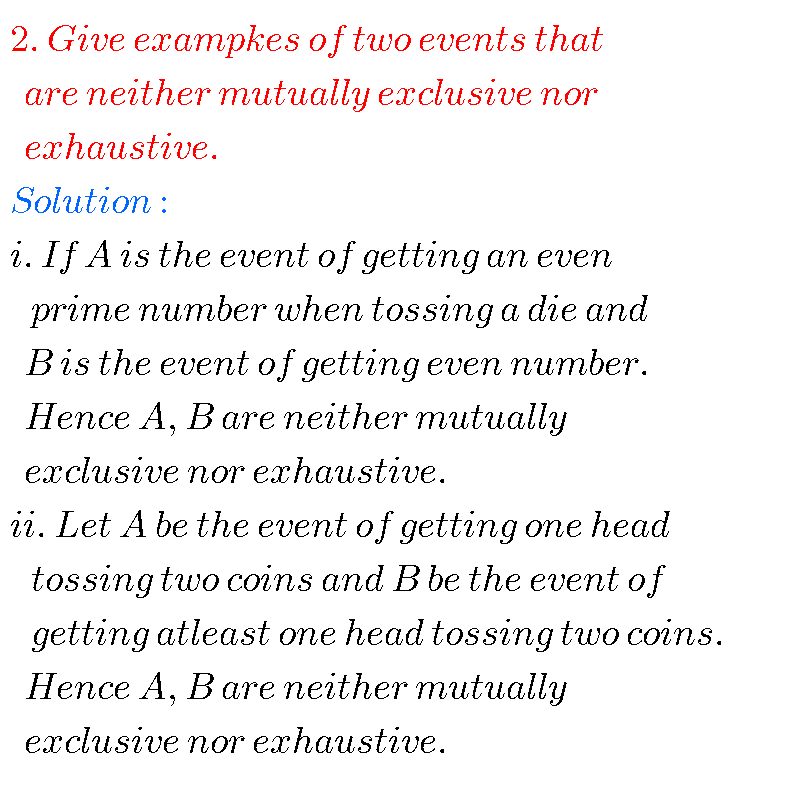## Inter maths solutions for Probability

Probability – Exercise 9(b)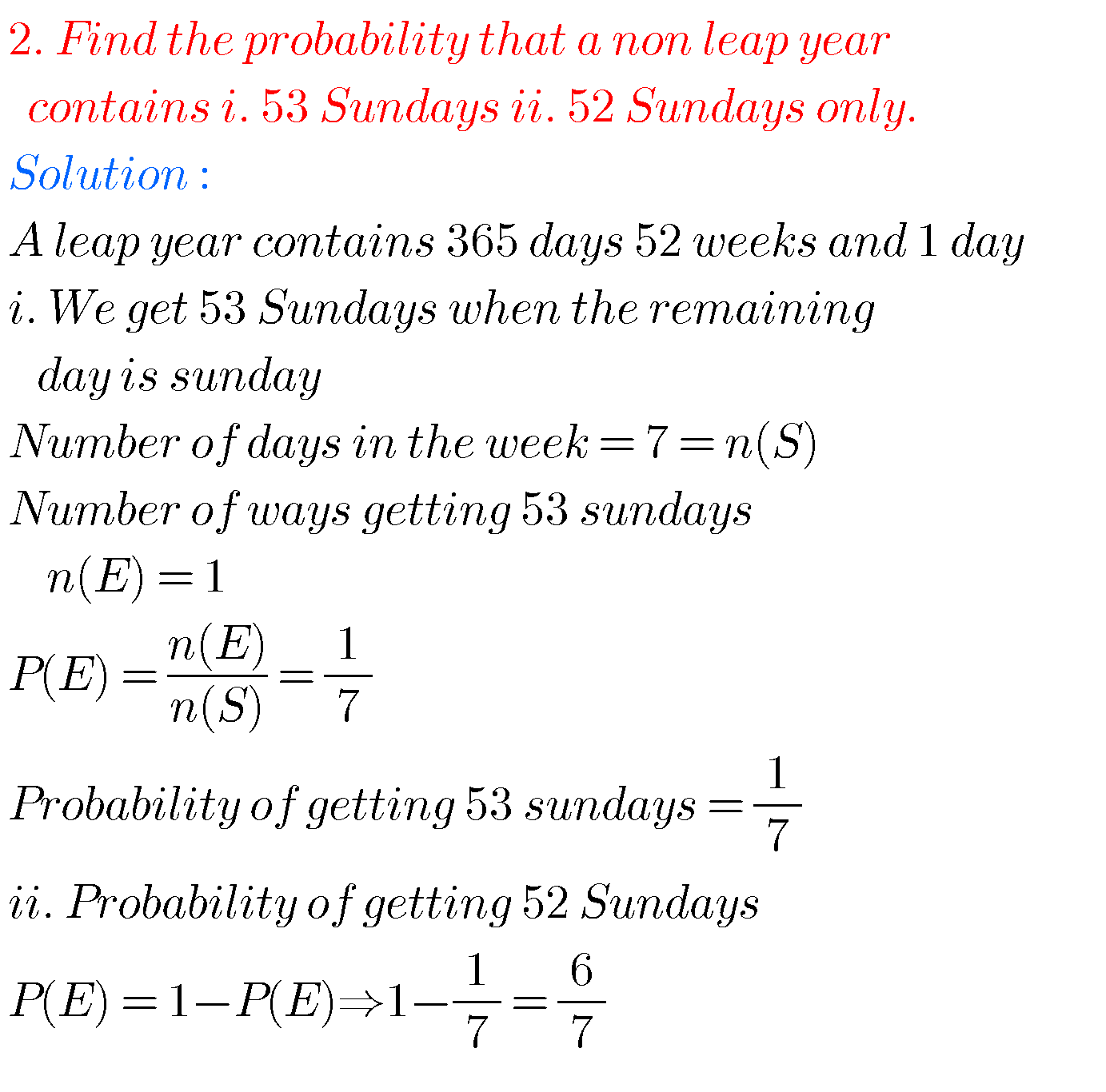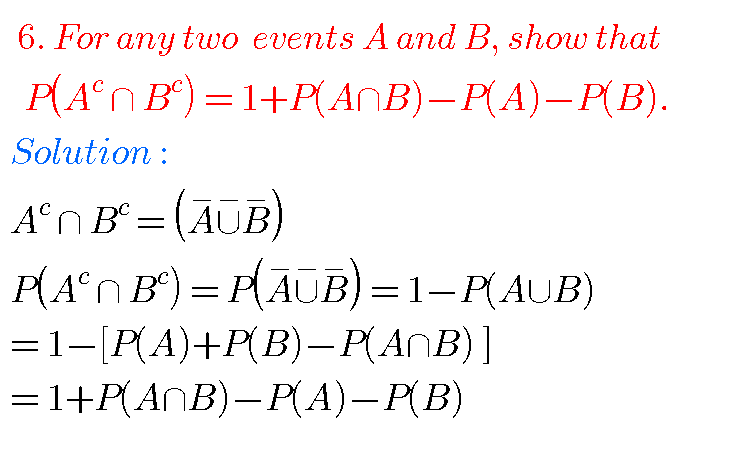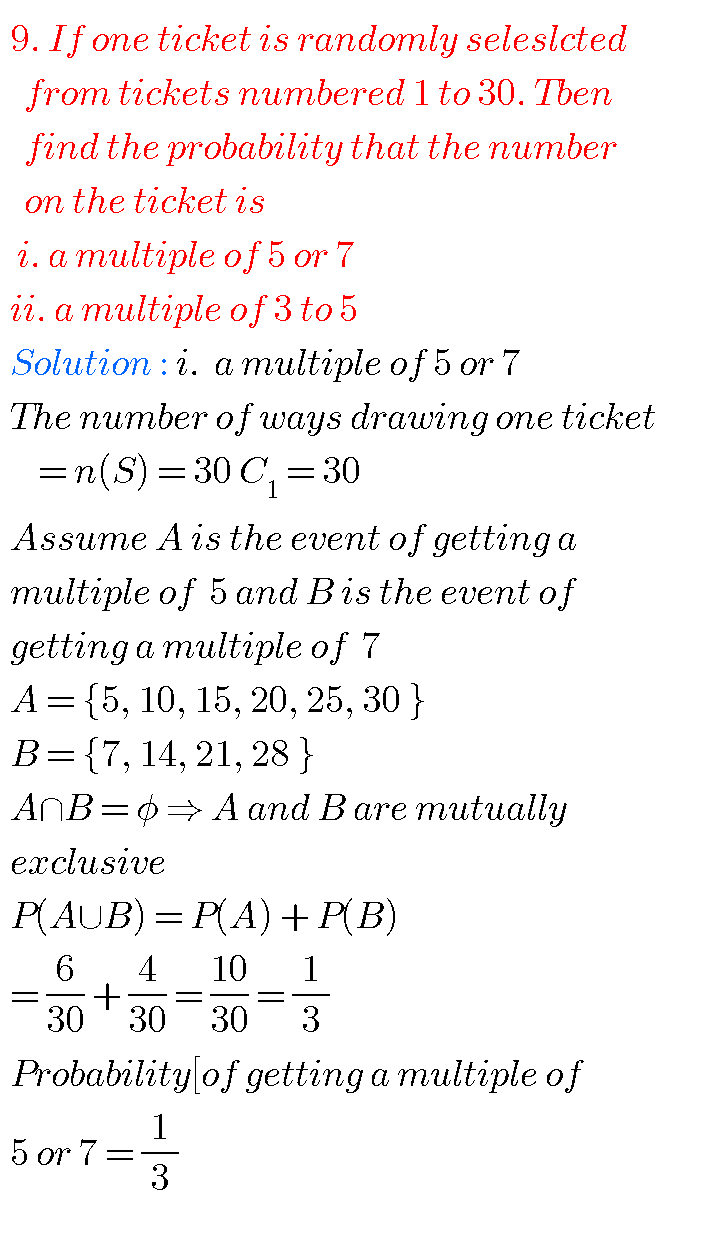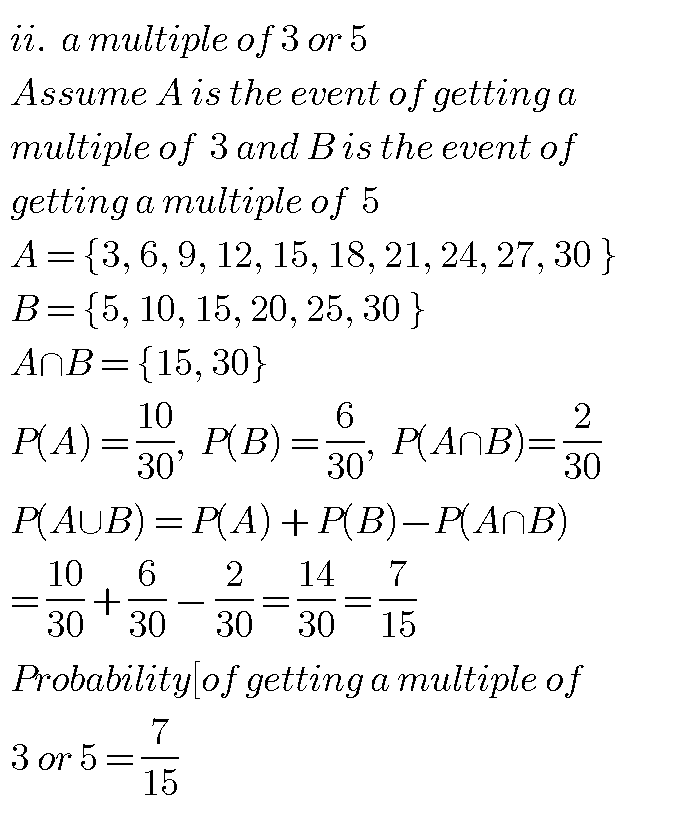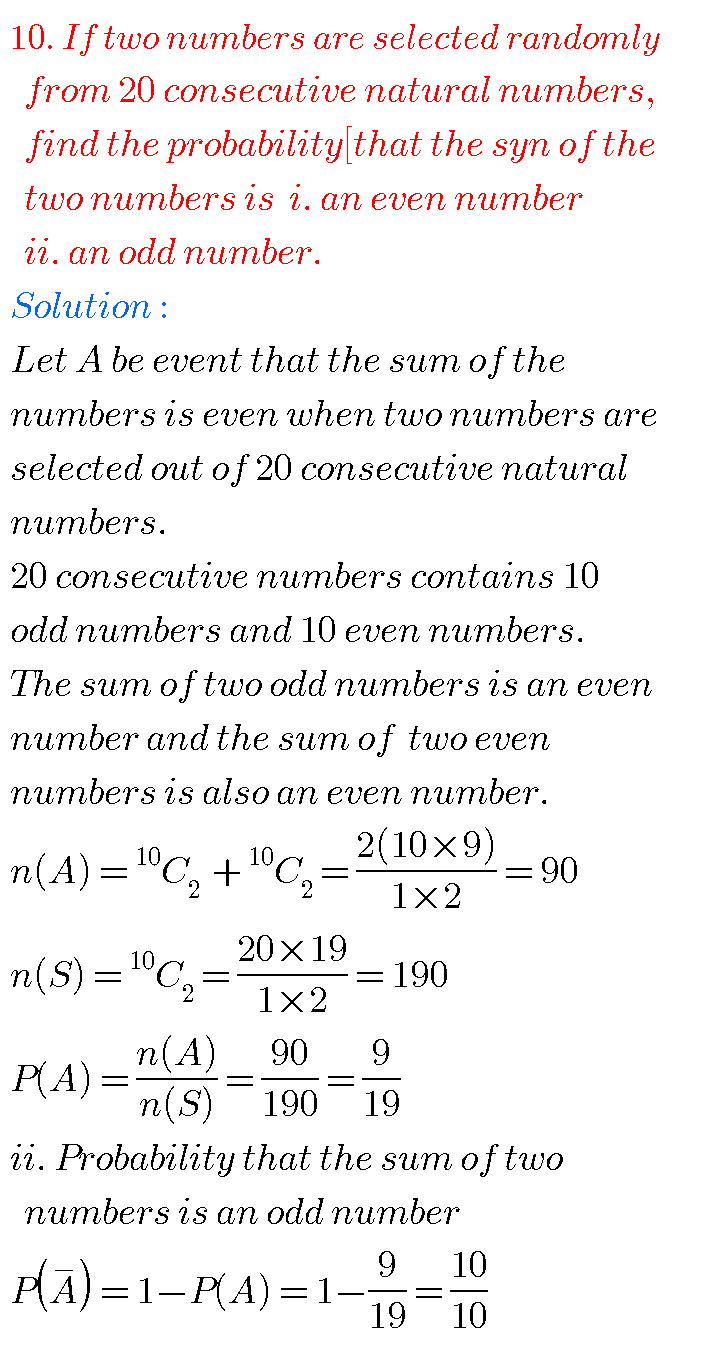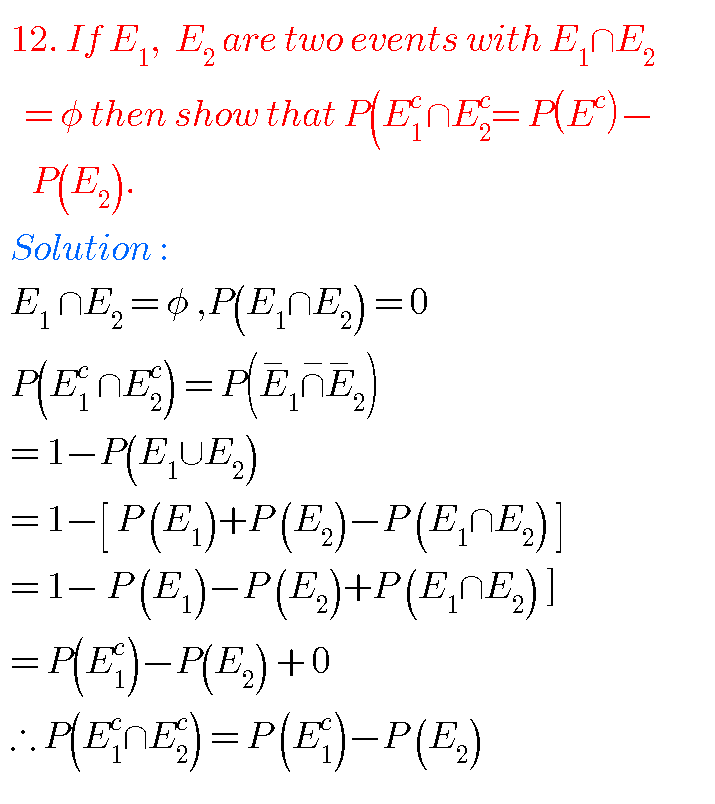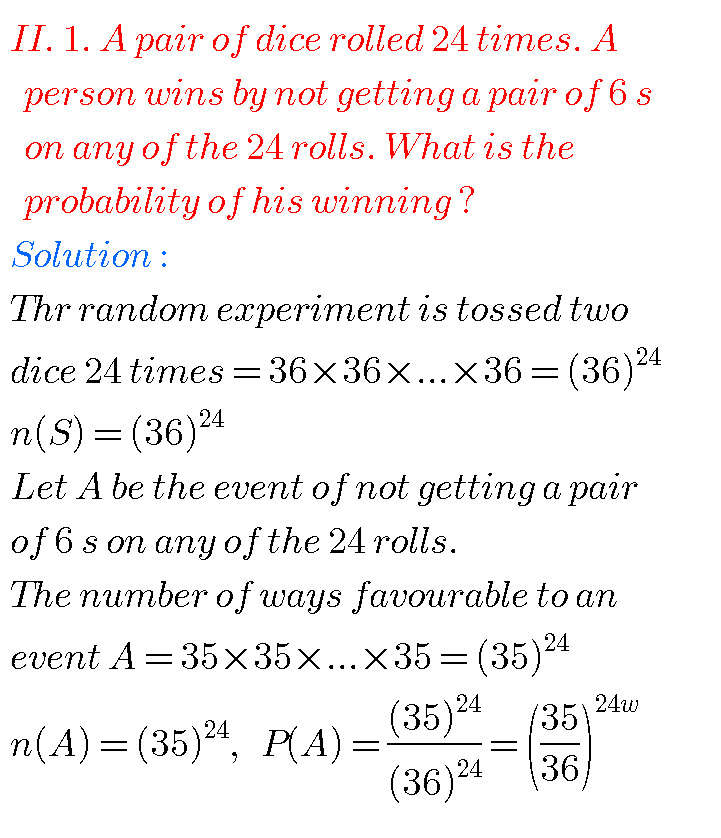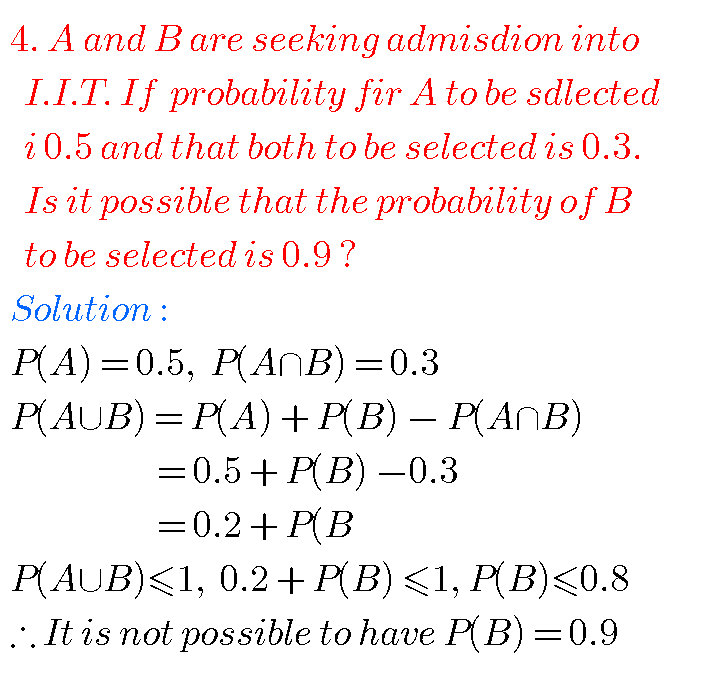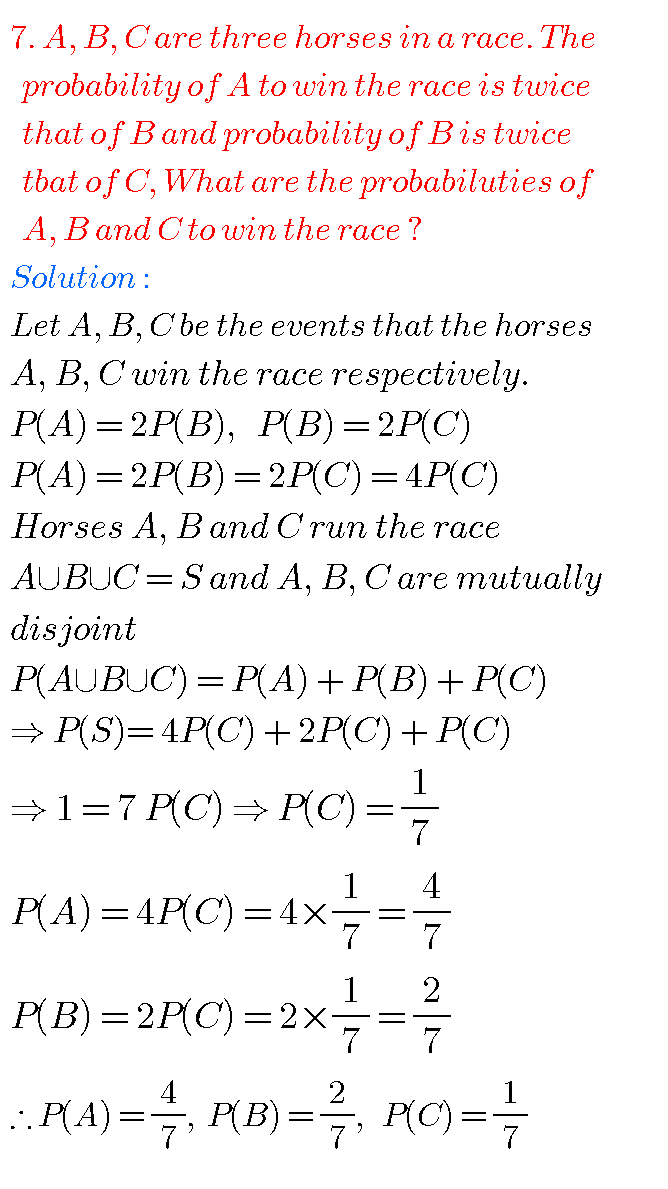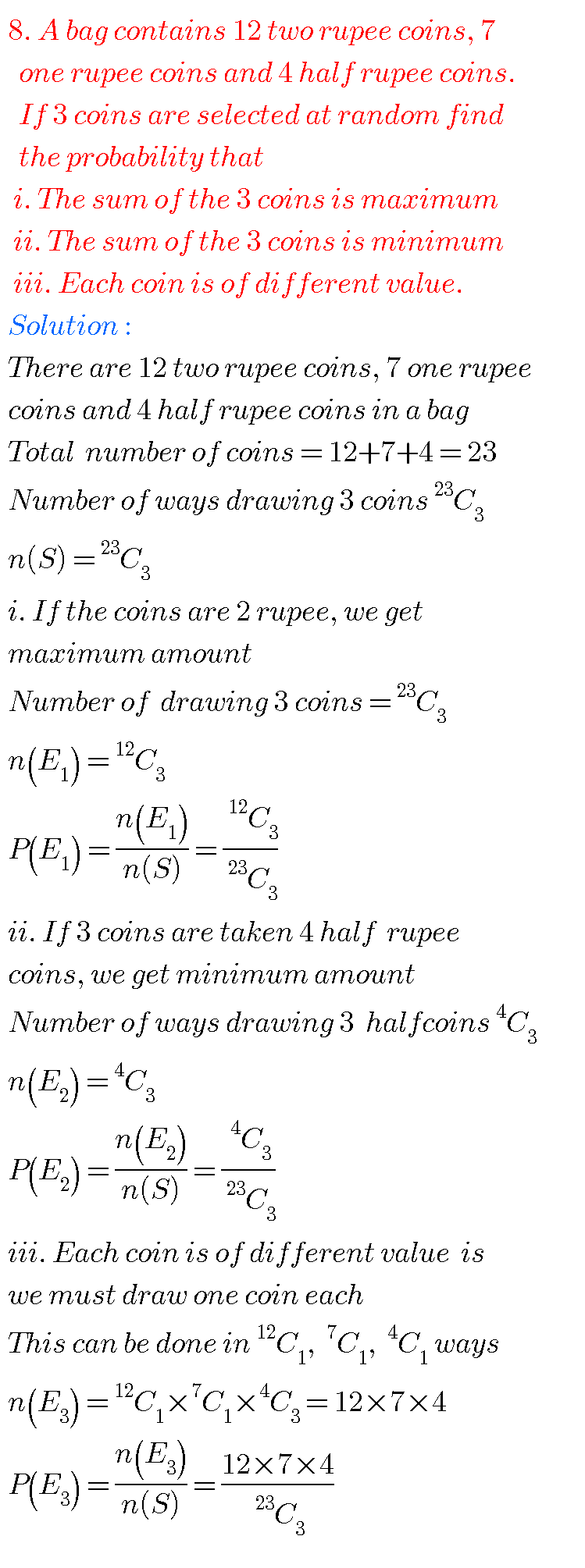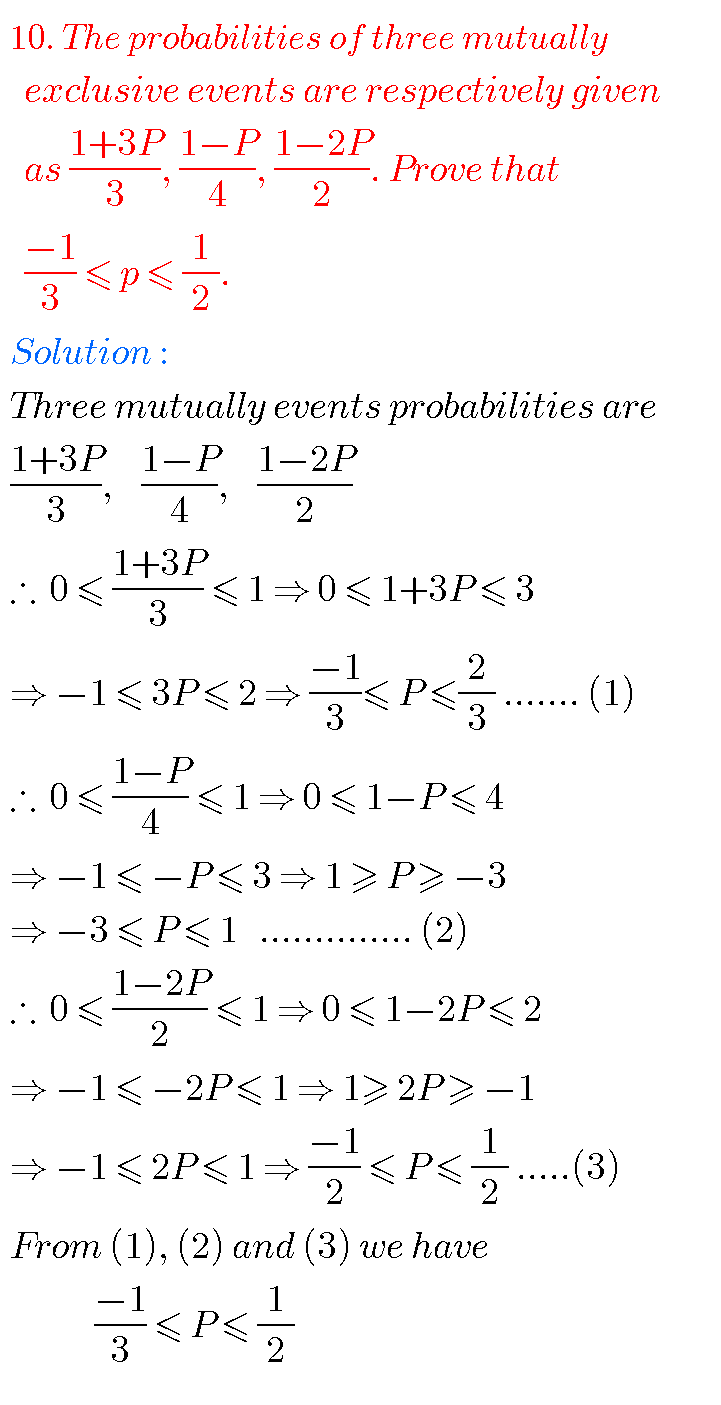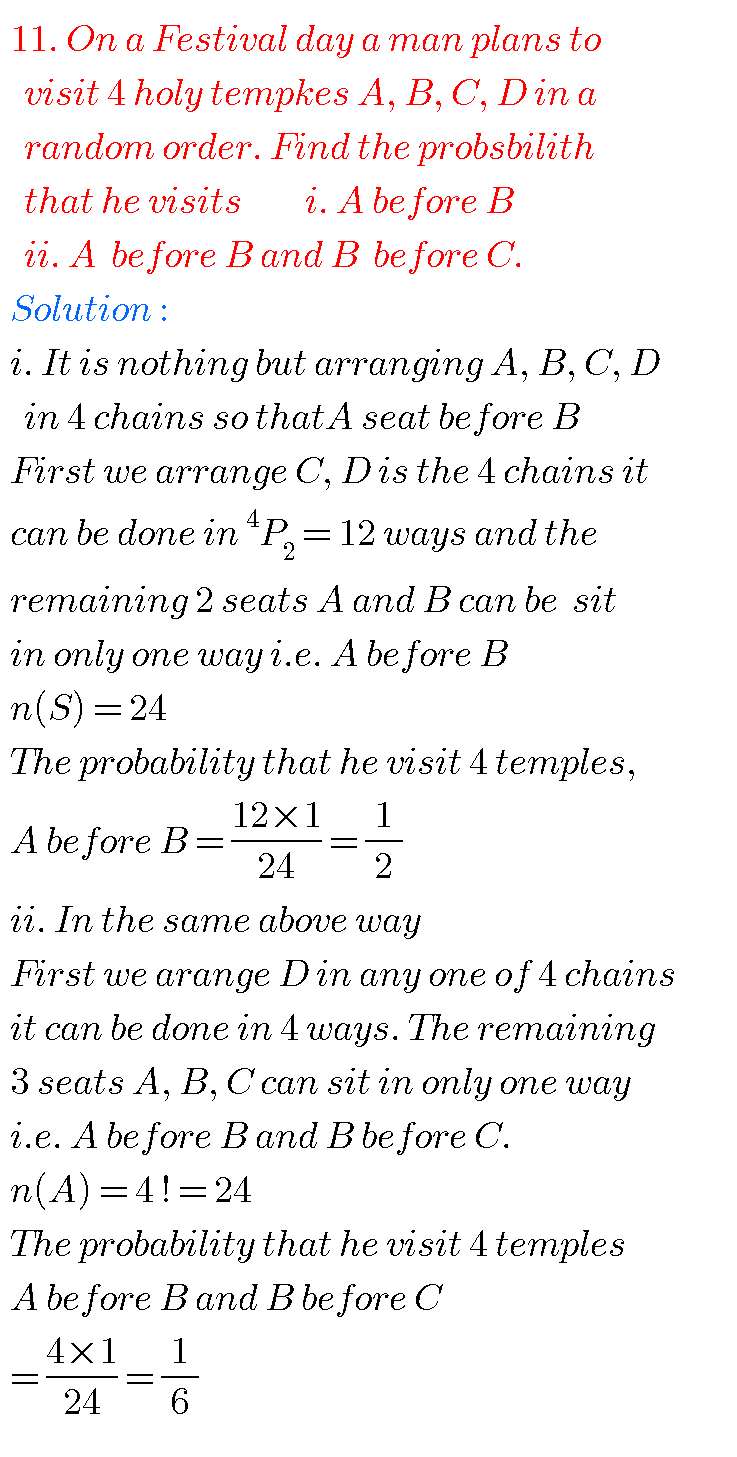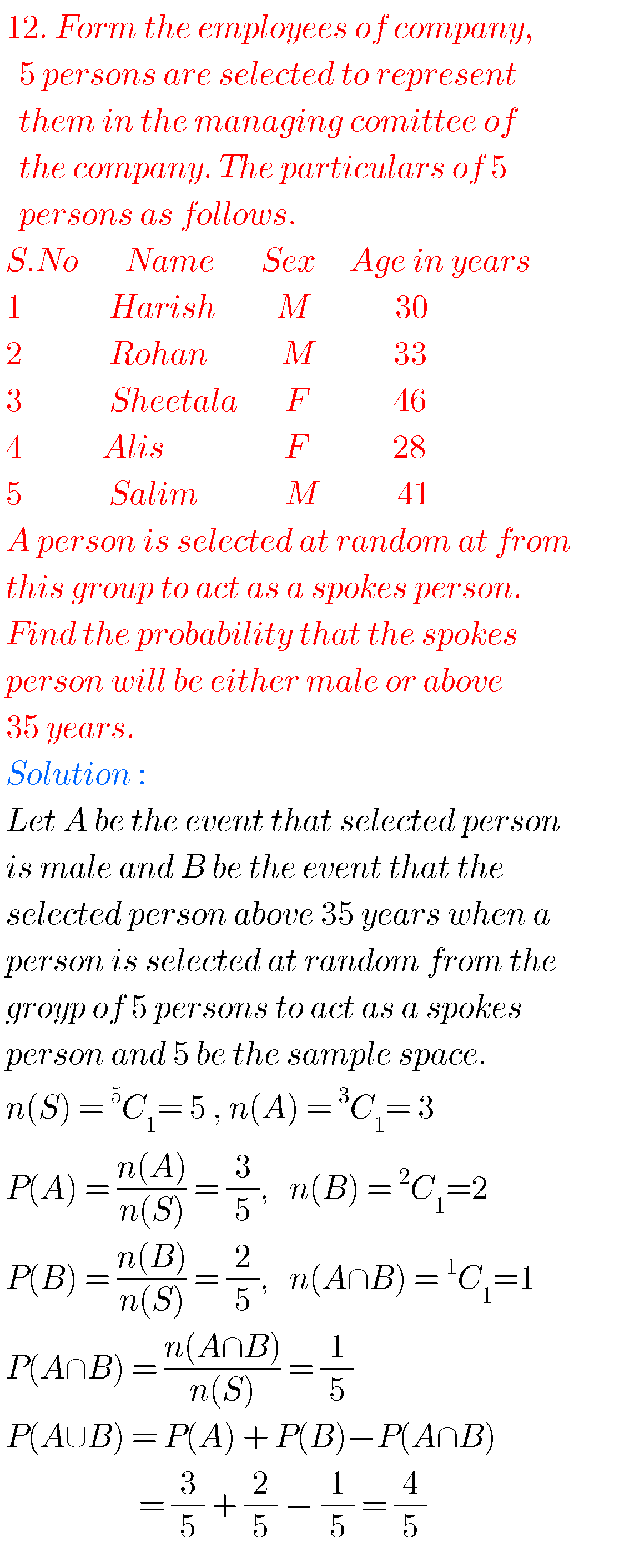### Solutions for Probability inter maths

Exercise 9(c)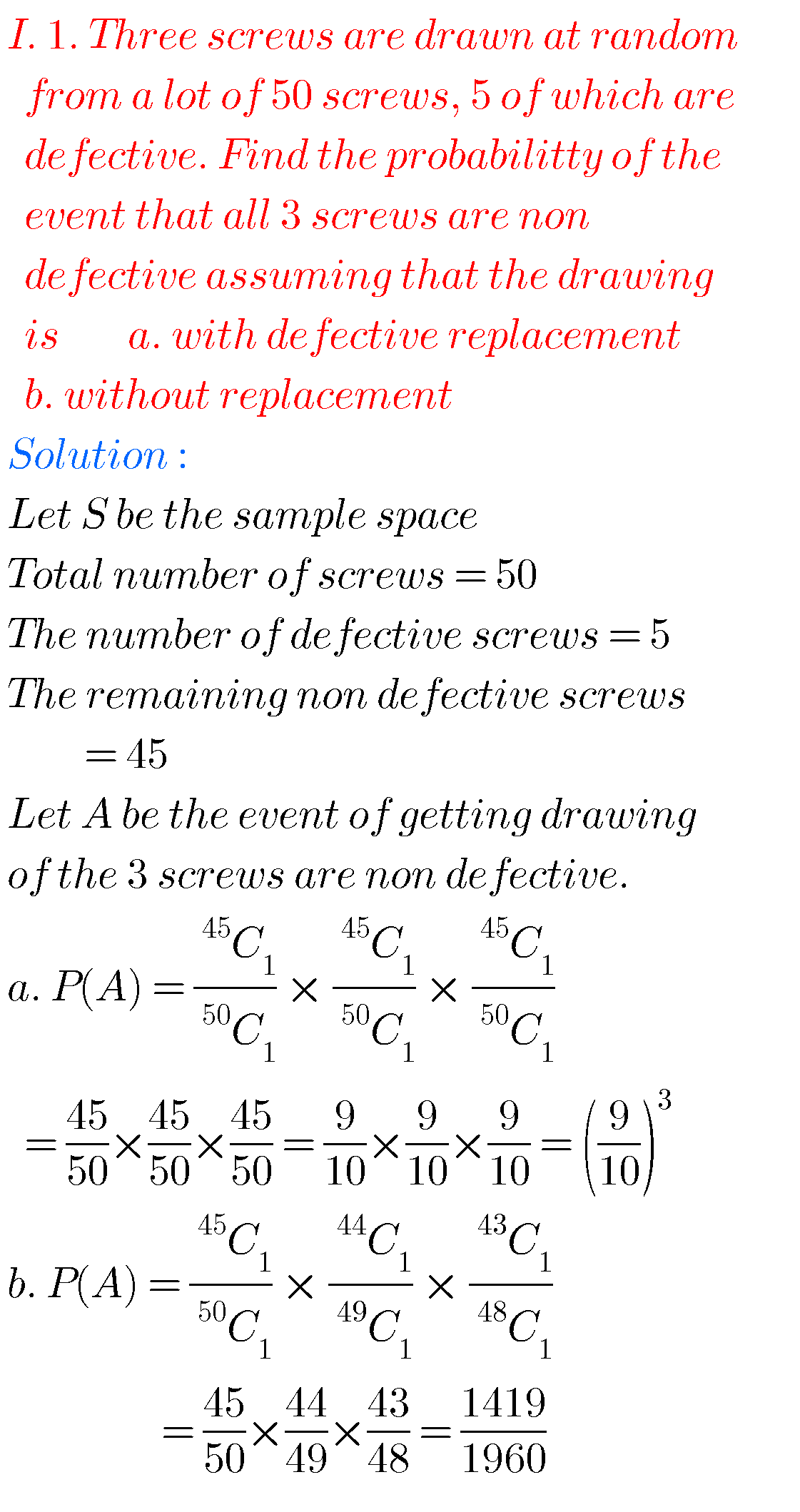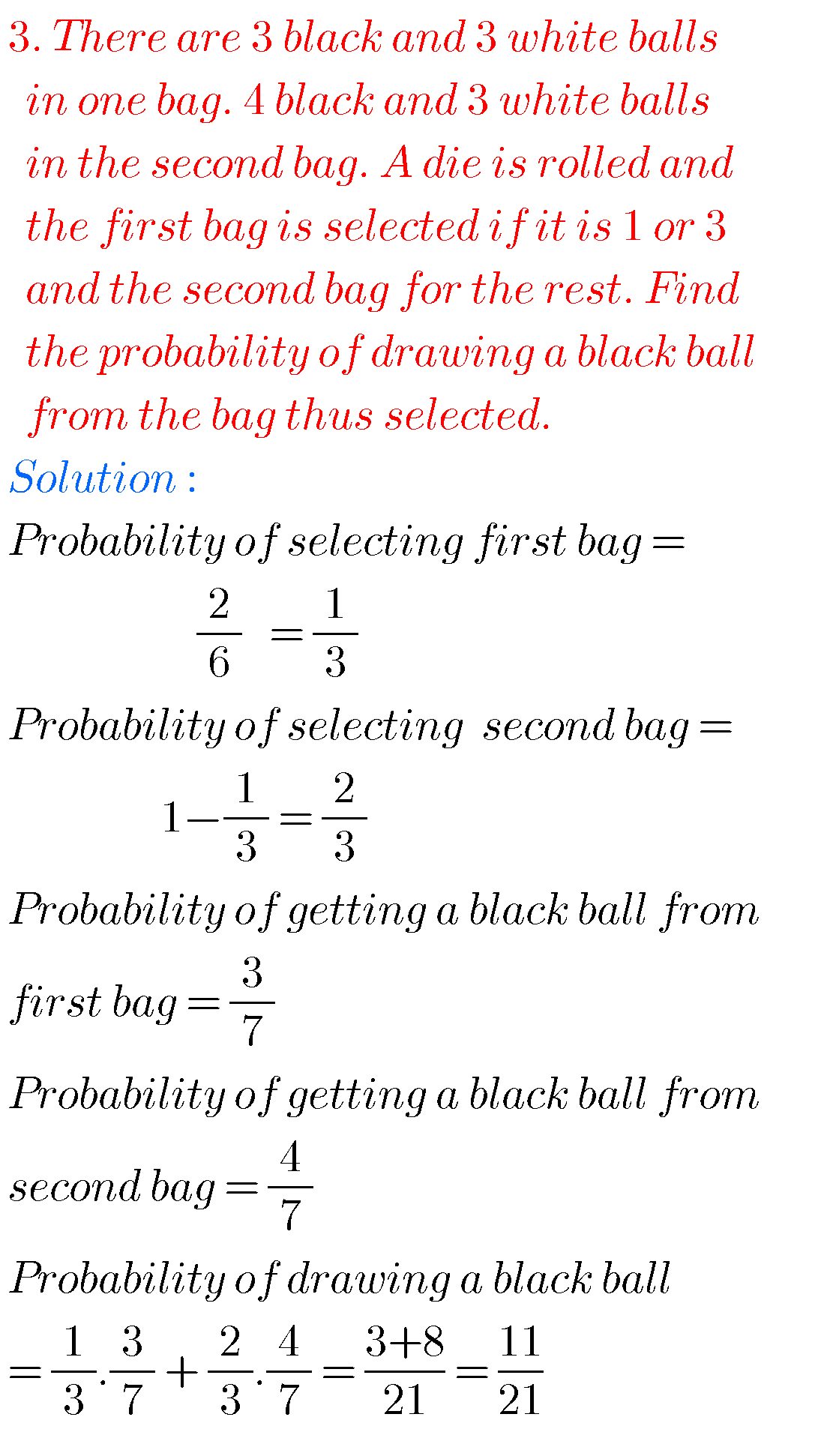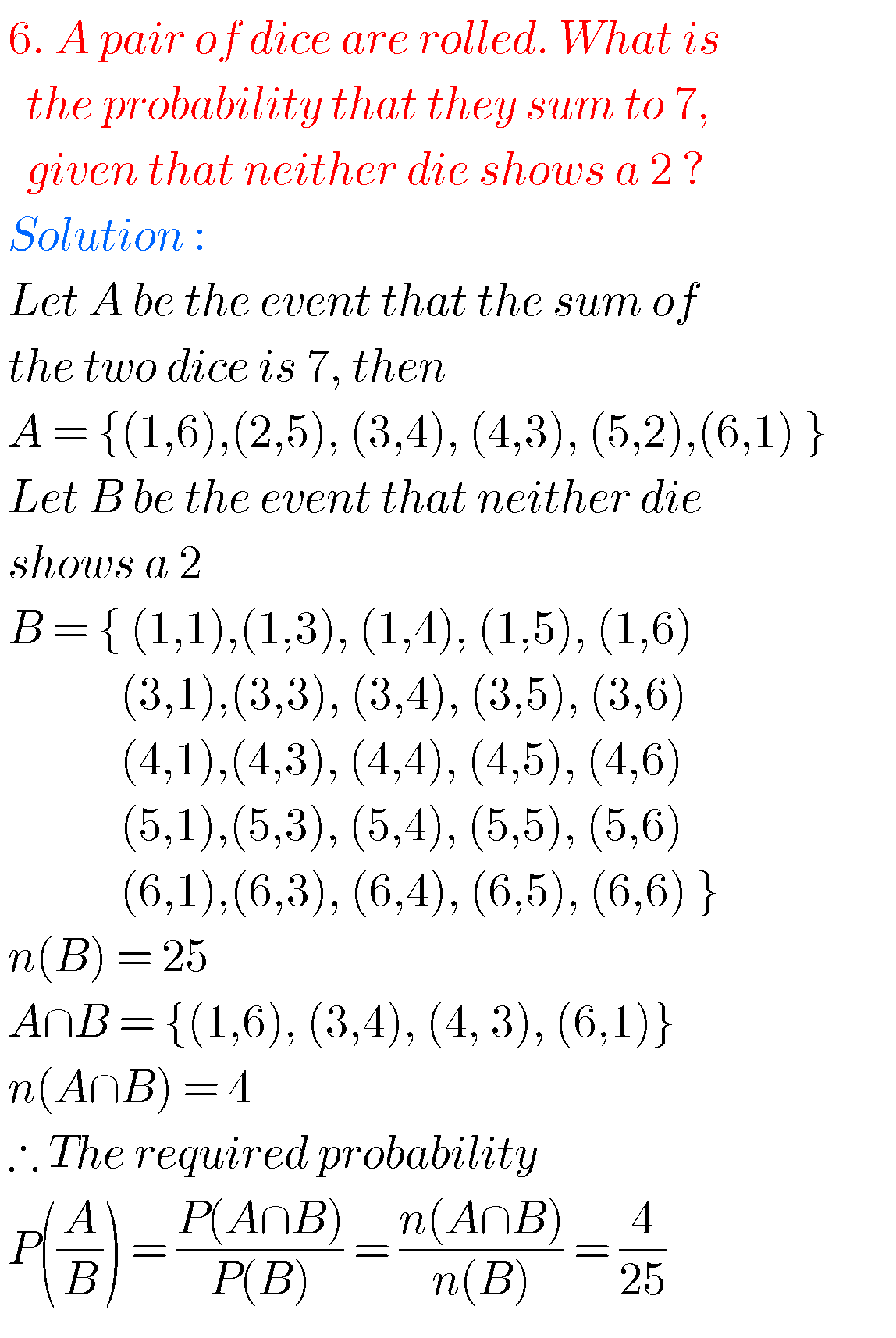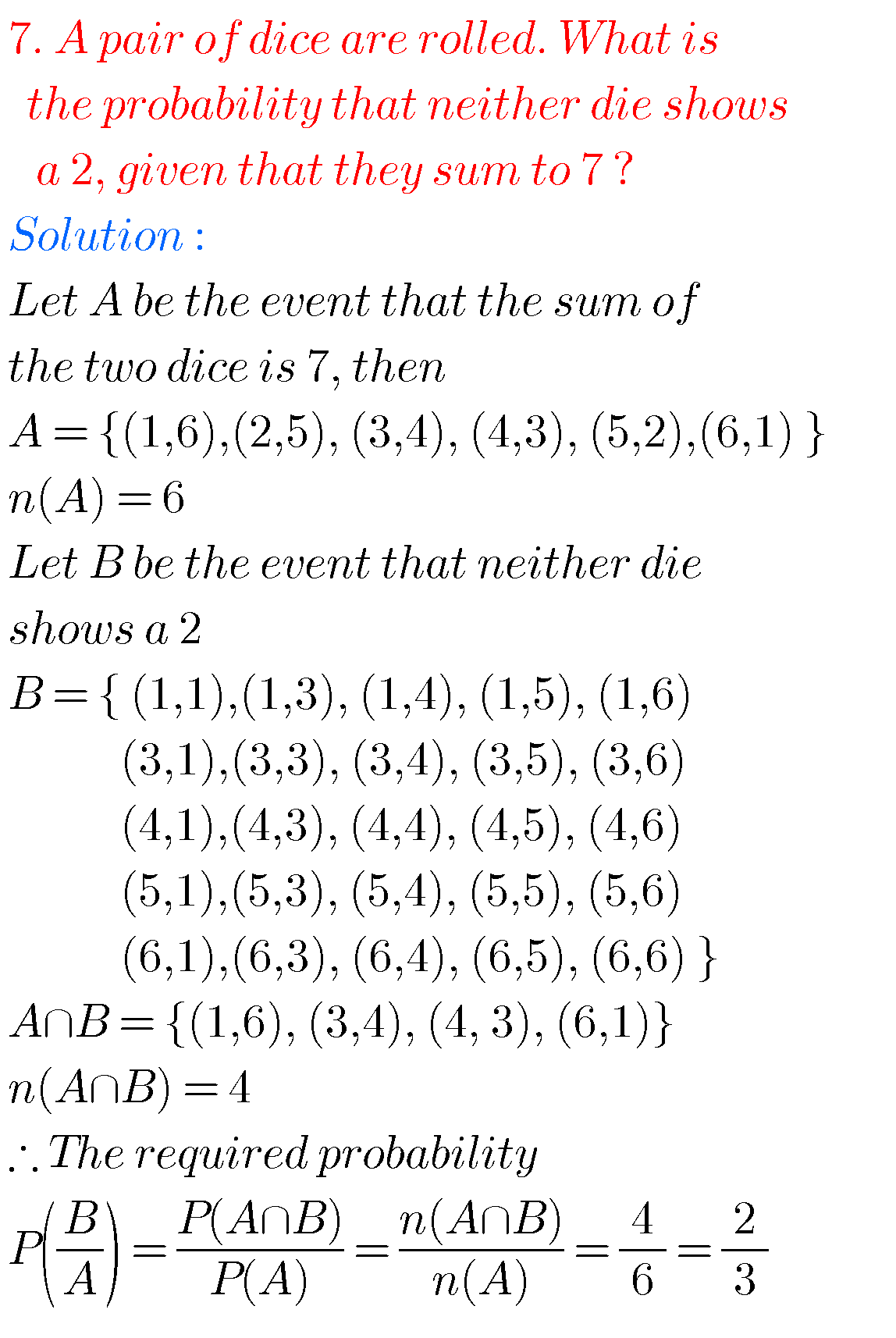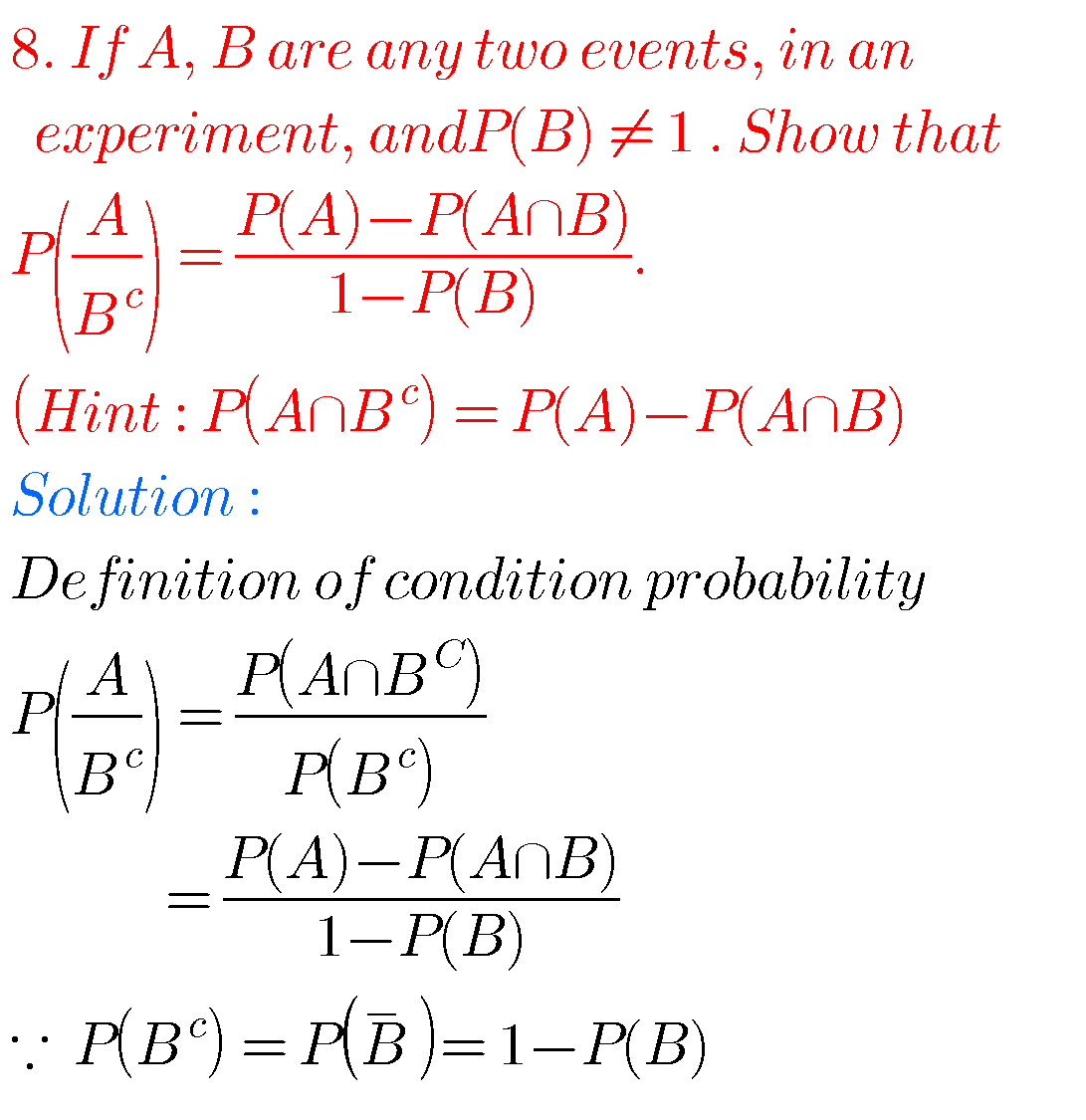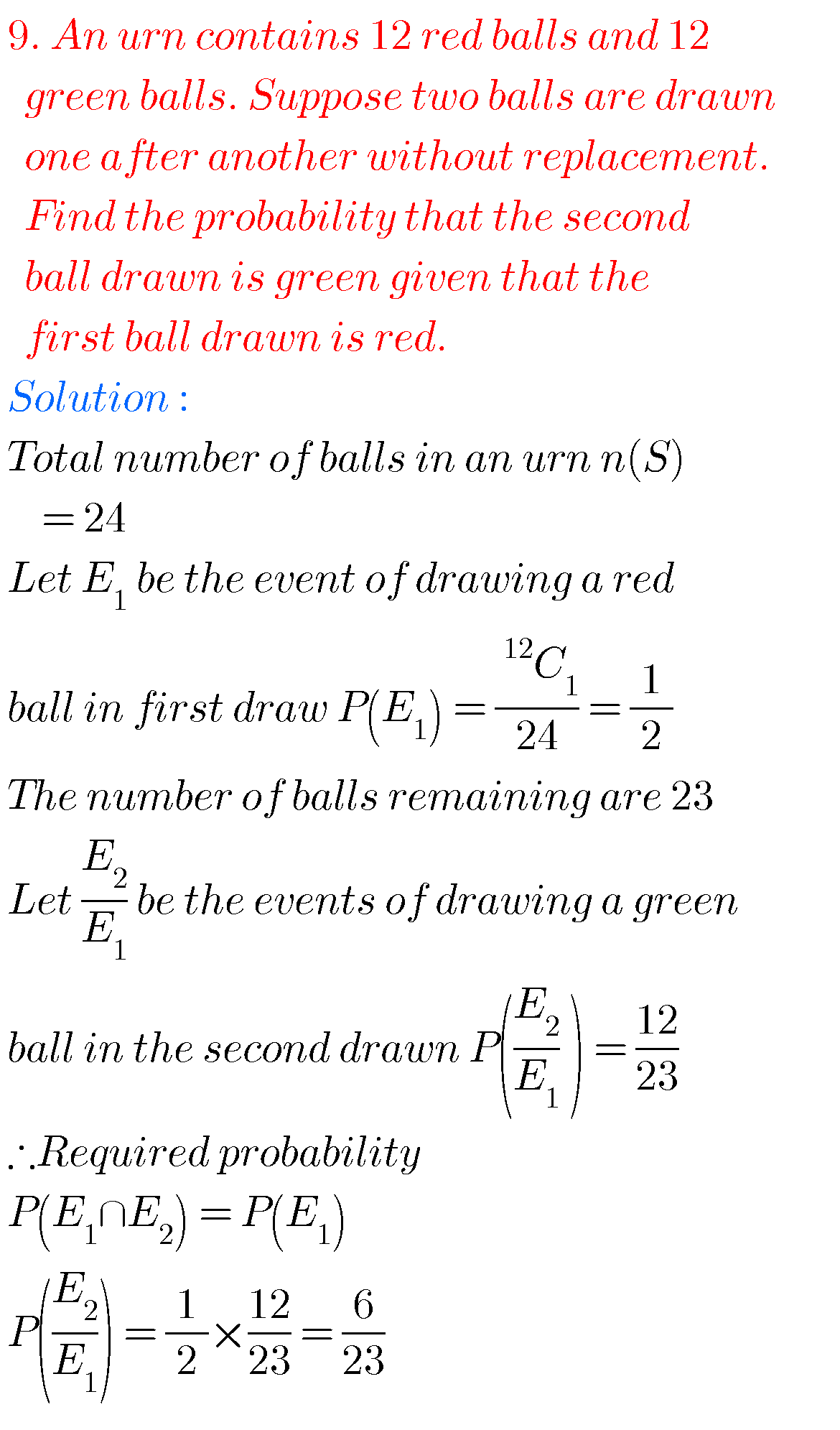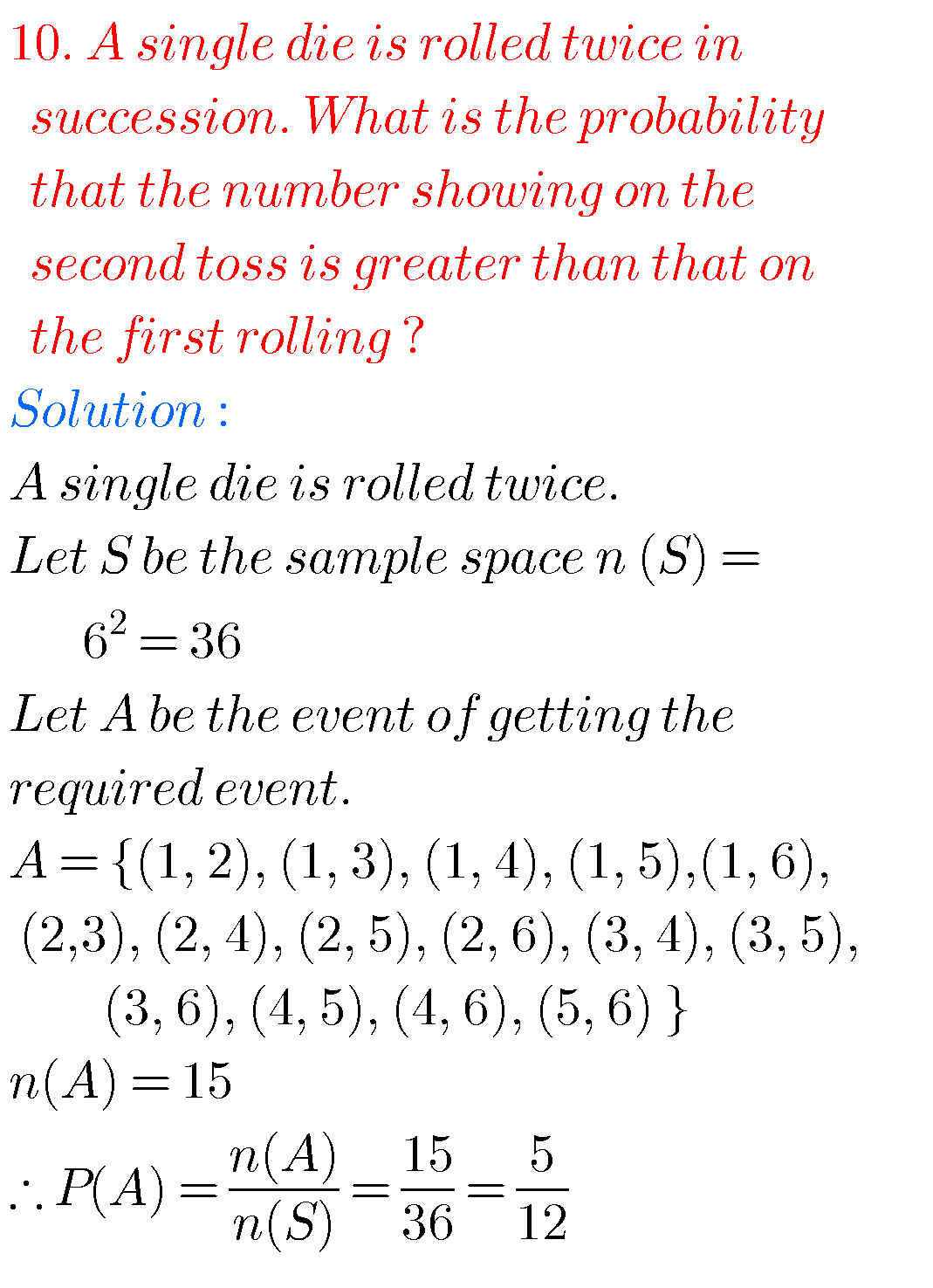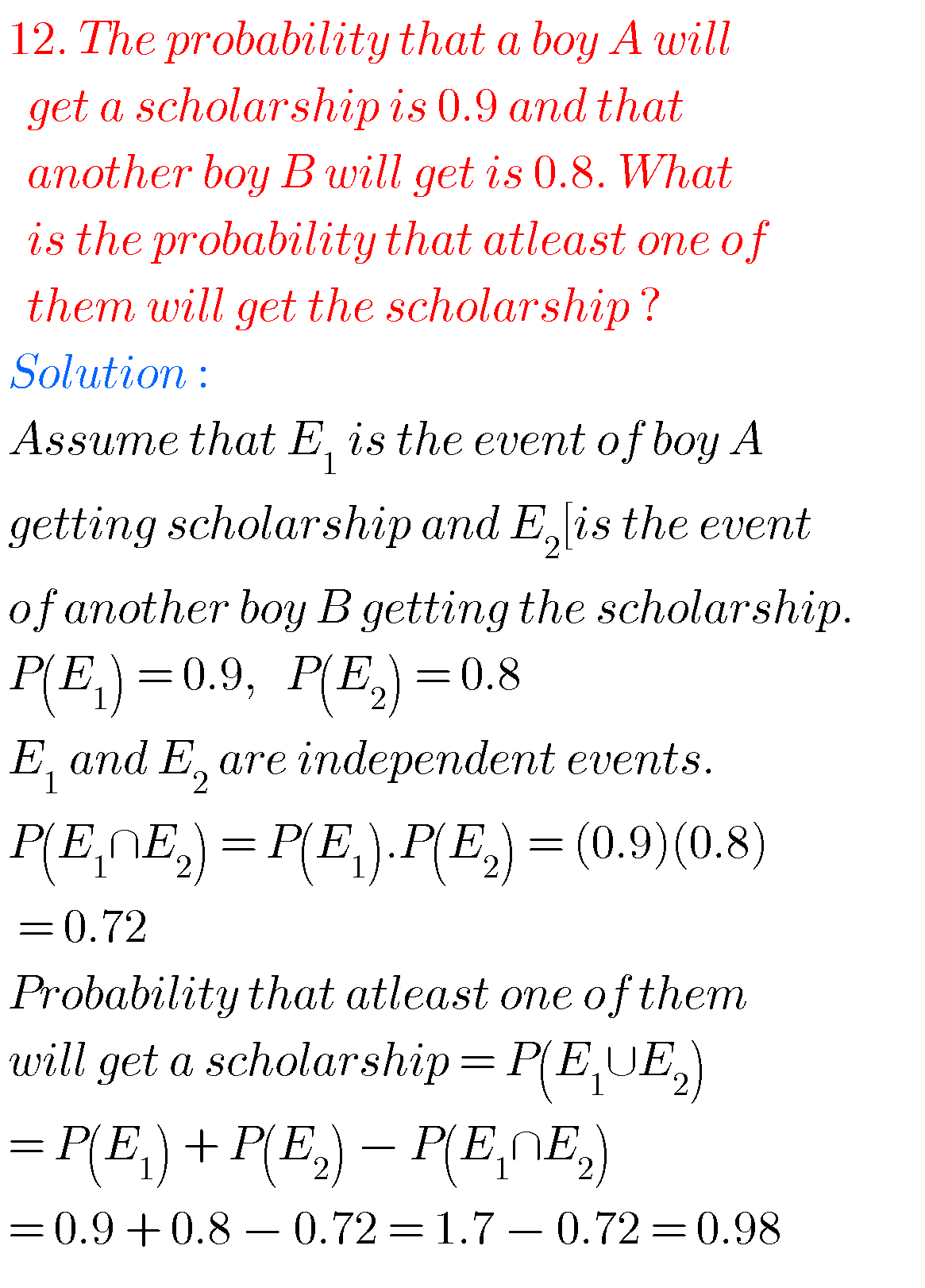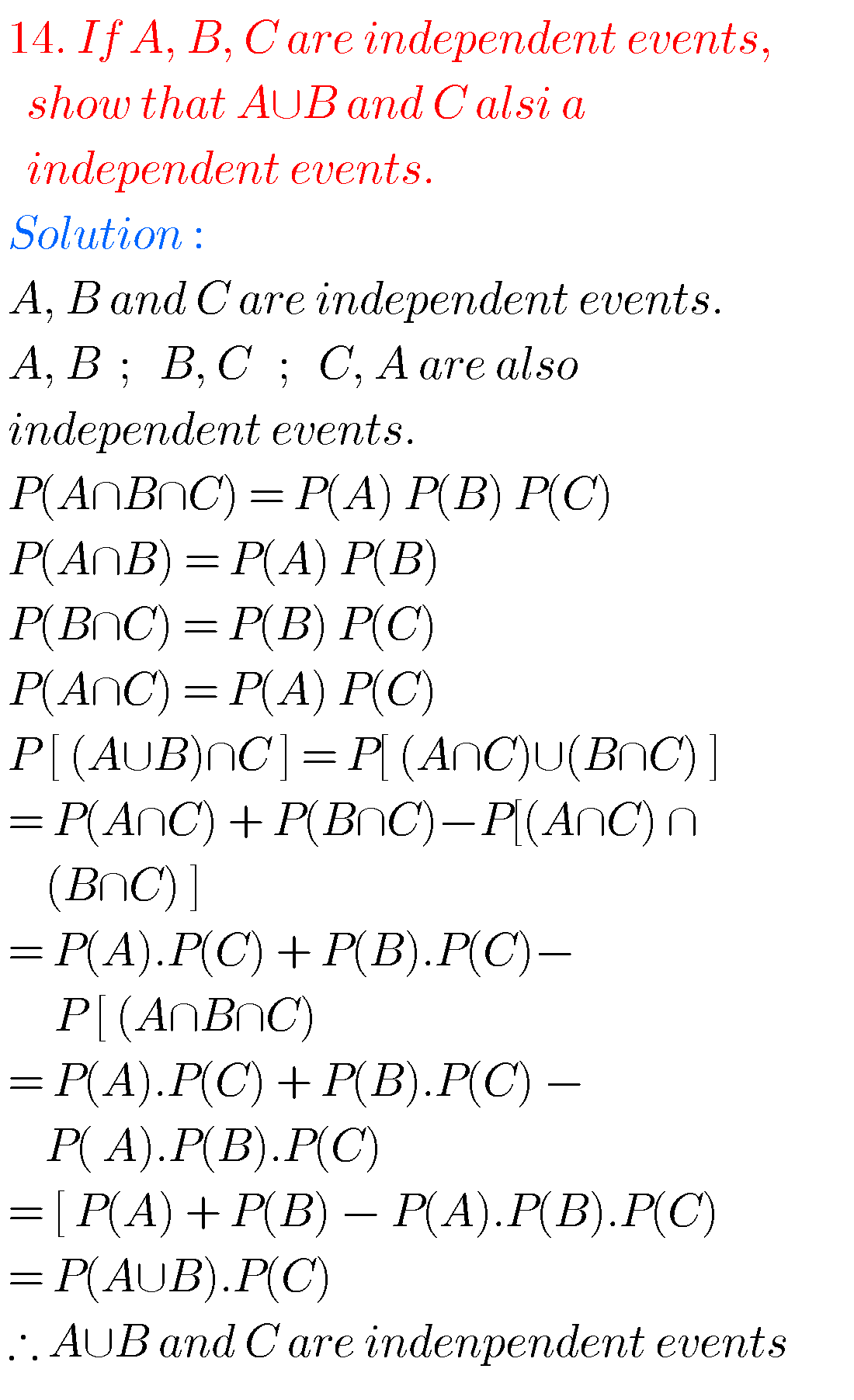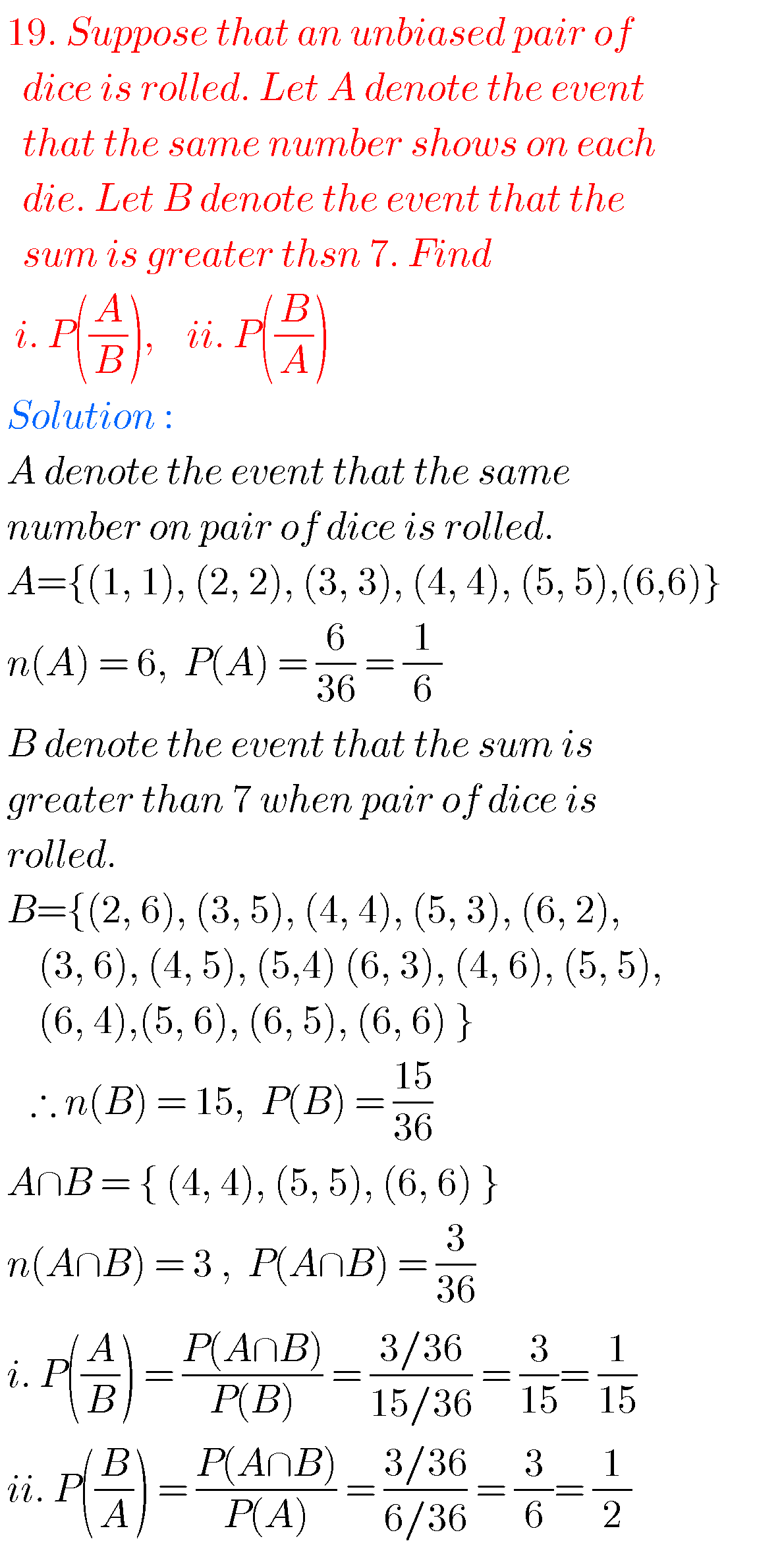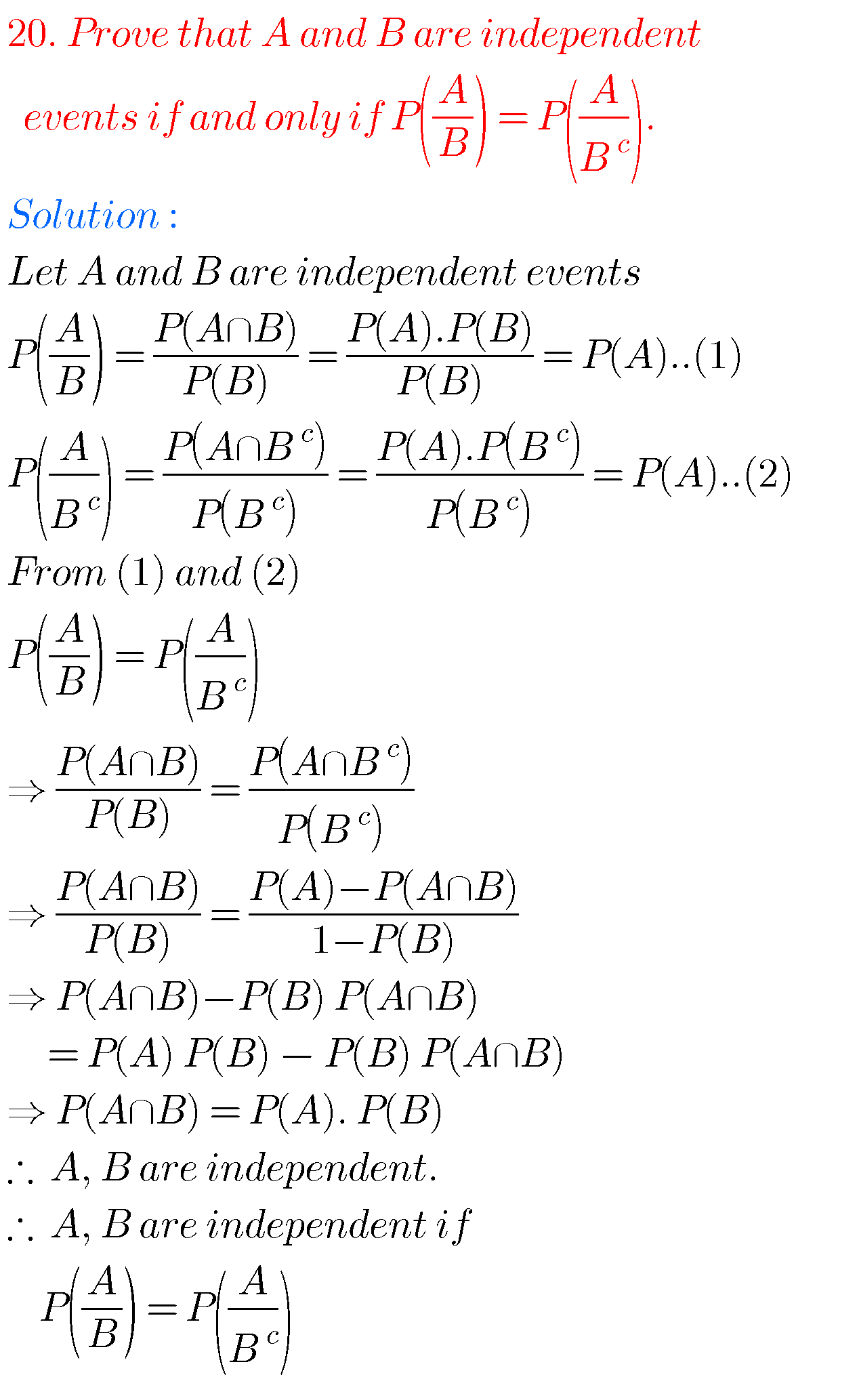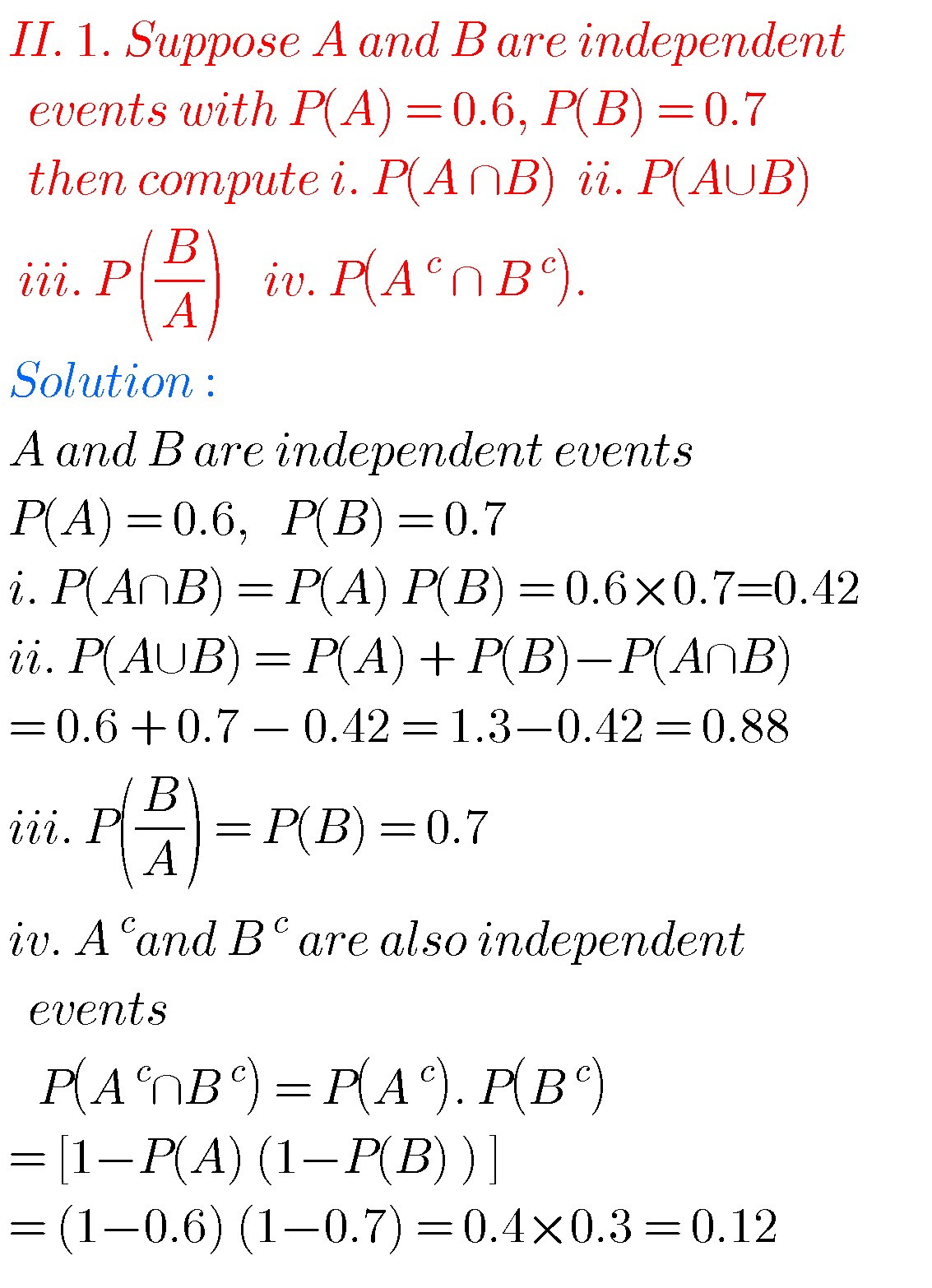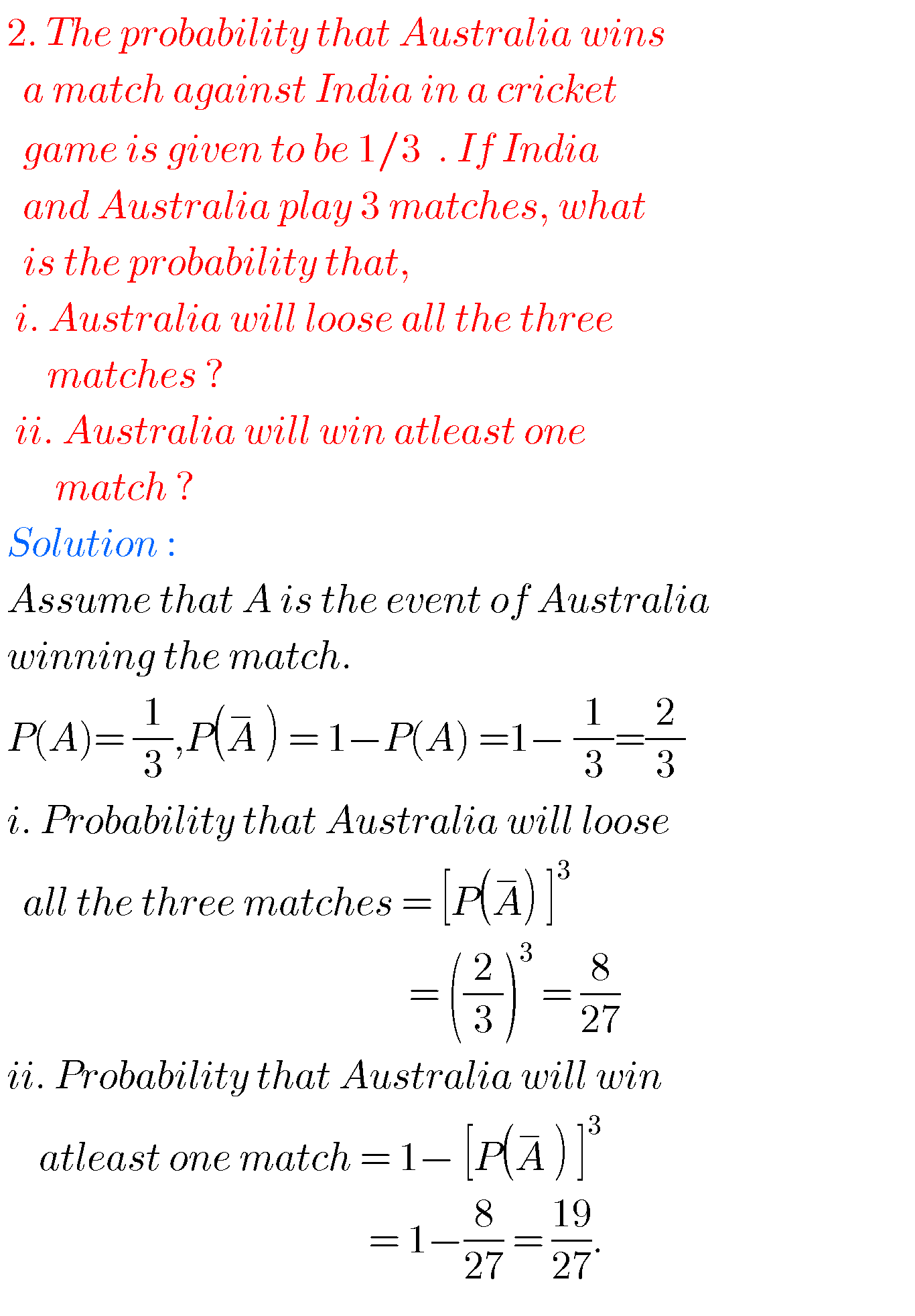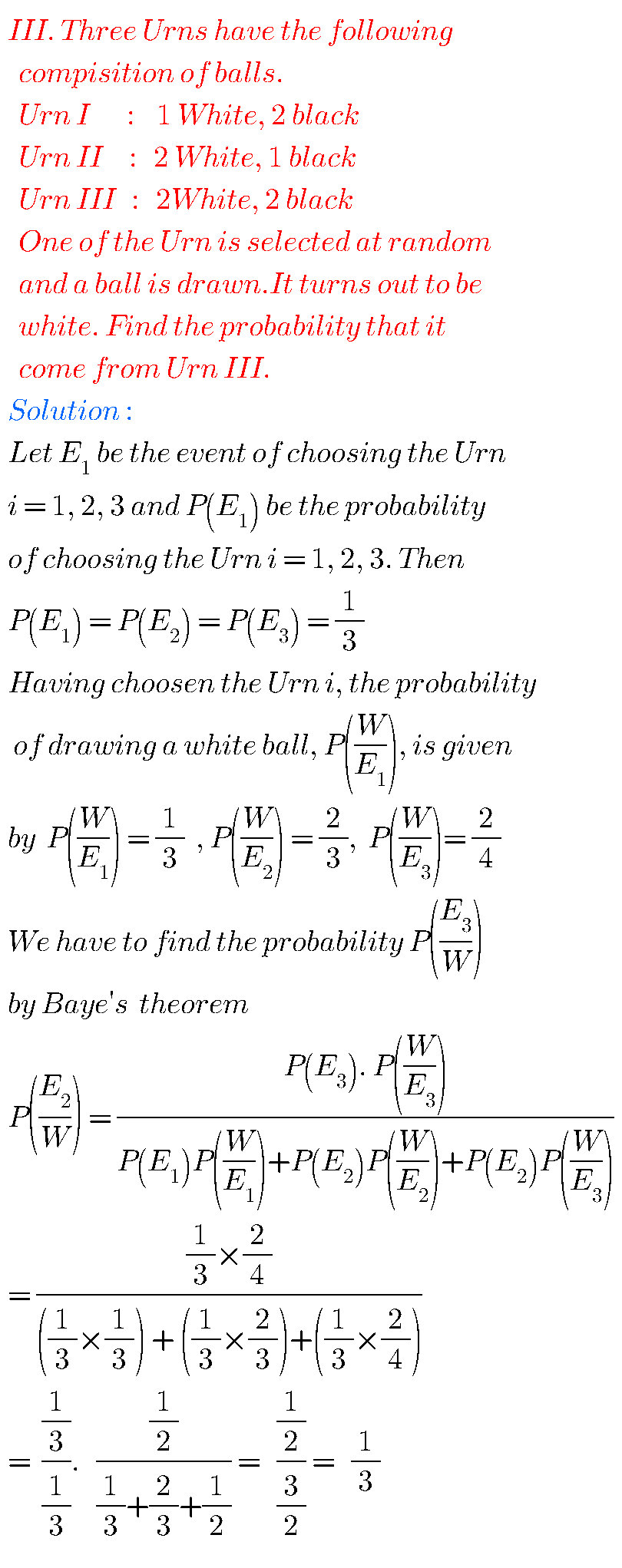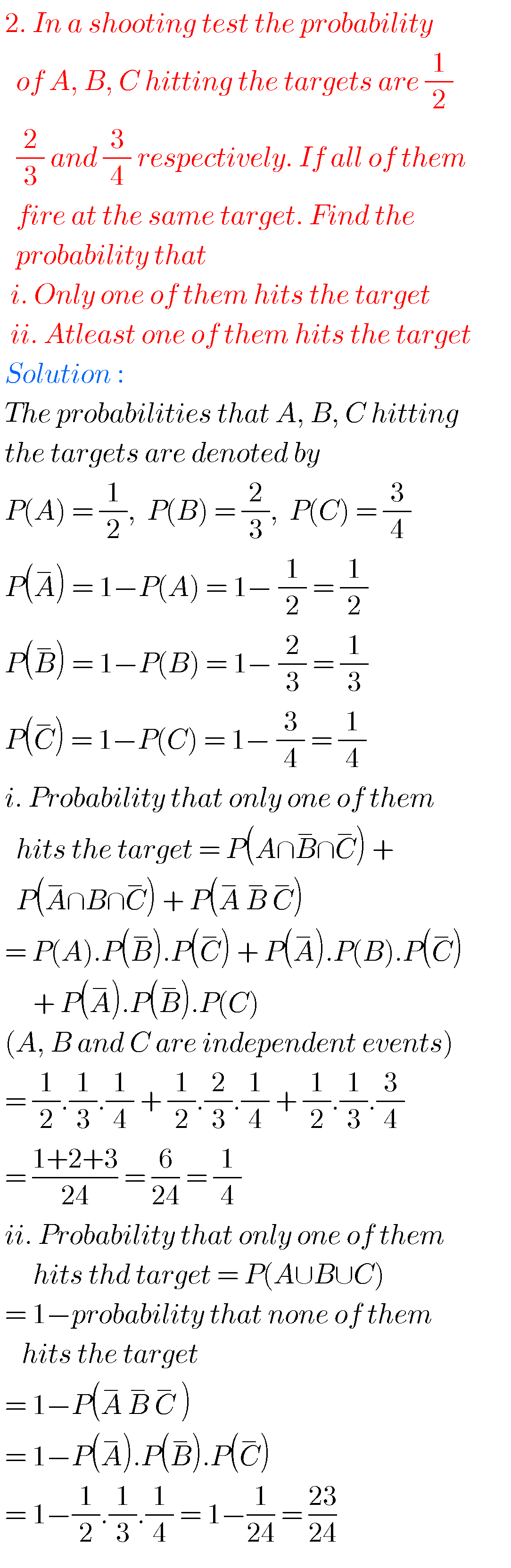Note : Observe the solutions annd try them in your own method.

Maths solutions for class 10 SSC

You can see the solutions for ssc maths

2. Sets

ou can see the solutions for text book Maths 1A

Functions

Exercise 1(a)

Exercise 1(b)

Exercise 1(c)

Mathematical Induction

Exercise 2(a)

Matrices

Exercise 3(a)

Exercise 3(b)

Exercise 3(c)

Exercise 3(d)

Exercise 3(e)

Exercise 3(f)

Exercise 3(g)

Exercise 3(h)

Exercise 3(i)

Exercise 4(a)

Exercise 4(b)

Product of Vectors

Exercise 5(a)

Exercise 5(b)

Exercise 5(c)

Trigonometric Ratios up to Transformations

Exercise 6(a)

Exercise 6(b)

Exercise 6(c)

Exercise 6(d)

Exercise 6(e)

Exercise 6(f)

Trigonometric Equations

Exercise 7(a)

Inverse Trigonometric Equations

Exercise 8(a)

Hyperbolic Functions

Exercise 9(a)

Properties of Triangles

Exercise 10(a)

Exercise 10(b)

Maths 1B

Locus

Exercise 1(a)

Transformation of axes

Exercise 2(a)

Three Dimensional Coordinates

Exercise 5(a)
Exercise 5(b)
Direction Cosines and Direction Ratios

Exercise 6(a)
Exercise 6(b)
The Plane

Exercise 7(a)
Limits and continuity

Exercise 8(a)

Exercise 8(b)